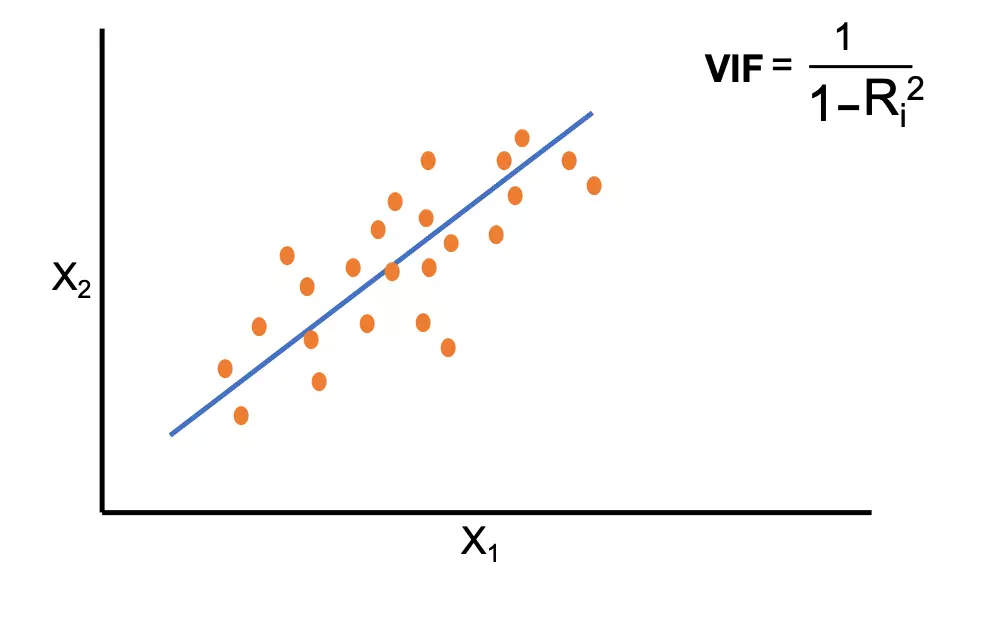# Multicollinearity and variance inflation factor (VIF) in the regression model (with Python code)## Multicollinearity and Variance inflation factor (VIF)

• Multicollinearity refers to high correlation in more than two independent variables in the regression model (e.g. Multiple linear regression). Similarly, collinearity refers to a high correlation between two independent variables.
• Multicollinearity can arise from poorly designed experiments (Data-based multicollinearity) or from creating new independent variables related to the existing ones (structural multicollinearity).
• Variance inflation factor (VIF) measures the degree of multicollinearity or collinearity in the regression model.
VIF is related to the R-squared, and its formula is,

$$VIF_i = \frac{1}{(1-R_i^2)}$$

Where $$R_i$$ = multiple correlation coefficient between $$X_i$$ and remaining independent variables (p-1)

i = 1,..., p (independent variables)

When $$R_i^2$$ = 0, there is a complete absence of multicollinearity, but when $$R_i^2$$ = 1, there is exact multicollinearity

## Multicollinearity diagnosis using variance inflation factor (VIF)

• VIF, tolerance indices (TIs), and correlation coefficients are useful metrics for multicollinearity detection.
• VIF range for assessing the multicollinearity is given as,
VIF value Diagnosis
1 Complete absence of multicollinearity
1-2 Absence of strong multicollinearity
> 2 Presence of moderate to strong multicollinearity

Note: There is no universal agreement of VIF values for multicollinearity detection. The VIF > 5 or VIF > 10 indicates strong multicollinearity, but VIF < 5 also indicates multicollinearity. It is advisable to have VIF < 2.

• VIF can detect multicollinearity, but it does not identify independent variables that are causing multicollinearity. Here, correlation analysis is useful for detecting highly correlated independent variables.

## Why multicollinearity is problematic in regression analysis?

• The efficiency of regression analysis largely depends on the correlation structure of the independent variables. The multicollinearity causes inaccurate results of regression analysis.
• If there is multicollinearity in the regression model, it leads to the biased and unstable estimation of regression coefficients, increases the variance and standard error of coefficients, and decreases the statistical power.
• For example, if the correlation between the two independent variables is greater than 0.9, it can significantly change the regression coefficients. Sometimes, change in the algebraic sign of regression coefficients can also occur.
• The multicollinearity is proportional to the estimation of regression coefficients. The higher the correlation between the independent variables, the higher will be the change in regression coefficients .

## How to fix multicollinearity?

• Increase the sample size
• Remove the highly correlated independent variables. If two independent variables are highly correlated, consider removing one of the variables. Removal of independent variables causing multicollinearity does not cause loss of information .
• Combine the highly correlated independent variables

## Example of diagnosis and correcting multicollinearity

• Now, you know multicollinearity is a serious issue in regression models. Here, we will discuss an example of multiple regression analysis to calculate the VIF using blood pressure data.
import pandas as pd
import statsmodels.api as sm
from statsmodels.stats.outliers_influence import variance_inflation_factor
X = df[['Age', 'Weight', 'BSA', 'Dur', 'Pulse', 'Stress']]   # independent variables
y = df['BP']   # dependent variables
# fit the regression model
reg = sm.OLS(y, X).fit()
# get Variance Inflation Factor (VIF)
pd.DataFrame({'variables':X.columns[1:], 'VIF':[variance_inflation_factor(X.values, i+1) for i in range(len(X.columns[1:]))]})
variables       VIF
0       Age  1.762807
1    Weight  8.417035
2       BSA  5.328751
3       Dur  1.237309
4     Pulse  4.413575
5    Stress  1.834845

• In the above example, the independent variables weight, BSA, and pulse (VIF > 2) are highly correlated with some independent variables in the model.
• As VIF does not tell which pair of independent variables are correlated, you can run a correlation analysis to know which variables are highly correlated.
X = df[['Age', 'Weight', 'BSA', 'Dur', 'Pulse', 'Stress']]   # independent variables
# correlation analysis
X.corr()
# output
Age    Weight       BSA       Dur     Pulse    Stress
Age     1.000000  0.407349  0.378455  0.343792  0.618764  0.368224
Weight  0.407349  1.000000  0.875305  0.200650  0.659340  0.034355
BSA     0.378455  0.875305  1.000000  0.130540  0.464819  0.018446
Dur     0.343792  0.200650  0.130540  1.000000  0.401514  0.311640
Pulse   0.618764  0.659340  0.464819  0.401514  1.000000  0.506310
Stress  0.368224  0.034355  0.018446  0.311640  0.506310  1.000000

• As per pairwise correlation analysis, Weight is highly correlated with BSA (r > 0.8) and Pulse (r > 0.6). To remove the multicollinearity, drop the variables BSA and Pulse, and reanalyze the regression model.
X = df[['Age', 'Weight', 'Dur', 'Stress']]   # independent variables
y = df['BP']   # dependent variables
# Variance Inflation Factor (VIF)
pd.DataFrame({'variables':X.columns[1:], 'VIF':[variance_inflation_factor(X.values, i+1) for i in range(len(X.columns[1:]))]})  variables       VIF
0       Age  1.468245
1    Weight  1.234653
2       Dur  1.200060
3    Stress  1.241117

1. Yoo W, Mayberry R, Bae S, Singh K, He QP, Lillard Jr JW. A study of effects of multicollinearity in the multivariable analysis. International journal of applied science and technology. 2014 Oct;4(5):9.
2. Vatcheva KP, Lee M, McCormick JB, Rahbar MH. Multicollinearity in regression analyses conducted in epidemiologic studies.
Epidemiology (Sunnyvale, Calif.). 2016 Apr;6(2).
3. Kim JH. Multicollinearity and misleading statistical results. Korean journal of anesthesiology. 2019 Dec;72(6):558.
4. Daoud JI. Multicollinearity and regression analysis. InJournal of Physics: Conference Series 2017 Dec 1 (Vol. 949, No. 1, p. 012009). IOP Publishing.
5. Marcoulides KM, Raykov T. Evaluation of variance inflation factors in regression models using latent variable modeling methods. Educational and psychological measurement. 2019 Oct;79(5):874-82.
6. Detecting Multicollinearity Using Variance Inflation Factors

If you have any questions, comments or recommendations, please email me at reneshbe@gmail.com